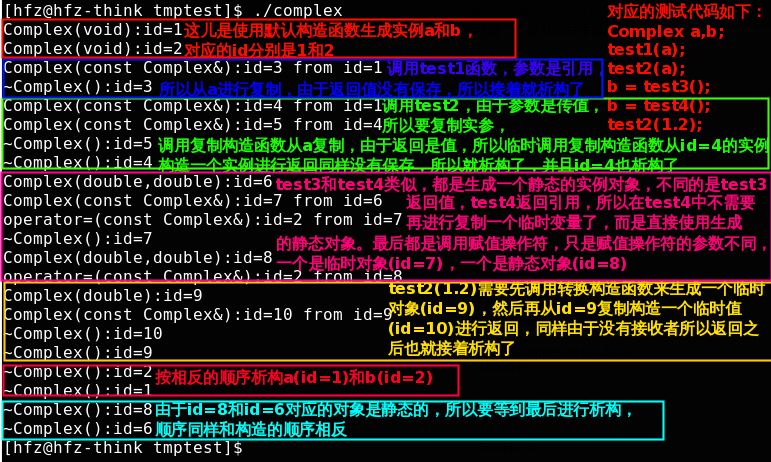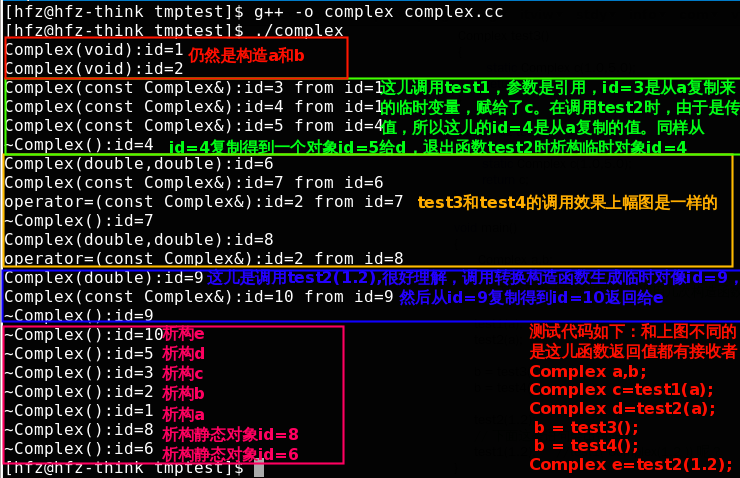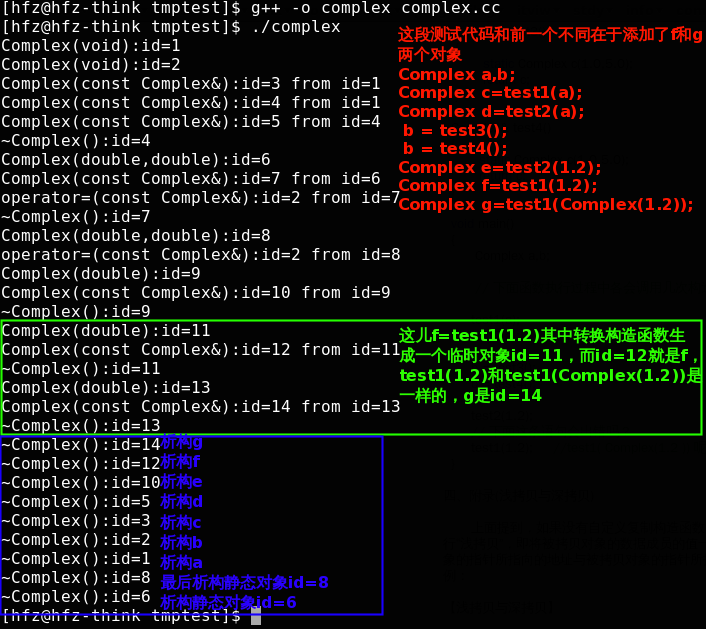# C++构造函数详解及显式调用构造函数

c++类的构造函数详解

class Counter
{
public:
// 类Counter的构造函数
// 特点：以类名作为函数名，无返回类型
Counter()
{
m_value = 0;
}
private:
// 数据成员
int m_value;
}
该类对象被创建时，编译系统对象分配内存空间，并自动调用该构造函数->由构造函数完成成员的初始化工作
eg:    Counter c1;
编译系统为对象c1的每个数据成员(m_value)分配内存空间，并调用构造函数Counter( )自动地初始化对象c1的m_value值设置为0

构造函数的作用：初始化对象的数据成员。

class Complex
{
private :
double    m_real;
double    m_imag;
public:
//    无参数构造函数
// 如果创建一个类你没有写任何构造函数,则系统会自动生成默认的无参构造函数，函数为空，什么都不做
// 只要你写了一个下面的某一种构造函数，系统就不会再自动生成这样一个默认的构造函数，如果希望有一个这样的无参构造函数，则需要自己显示地写出来
Complex(void)
{
m_real = 0.0;
m_imag = 0.0;
}
//    一般构造函数（也称重载构造函数）
// 一般构造函数可以有各种参数形式,一个类可以有多个一般构造函数，前提是参数的个数或者类型不同（基于c++的重载函数原理）
// 例如：你还可以写一个 Complex( int num)的构造函数出来
// 创建对象时根据传入的参数不同调用不同的构造函数
Complex(double real, double imag)
{
m_real = real;
m_imag = imag;
}
//    复制构造函数（也称为拷贝构造函数）
//    复制构造函数参数为类对象本身的引用，用于根据一个已存在的对象复制出一个新的该类的对象，一般在函数中会将已存在对象的数据成员的值复制一份到新创建的对象中
//    若没有显示的写复制构造函数，则系统会默认创建一个复制构造函数，但当类中有指针成员时，由系统默认创建该复制构造函数会存在风险，具体原因请查询 有关 “浅拷贝” 、“深拷贝”的文章论述
Complex(const Complex & c)
{
// 将对象c中的数据成员值复制过来
m_real = c.m_real;
m_imag    = c.m_imag;
}
// 类型转换构造函数，根据一个指定的类型的对象创建一个本类的对象
//需要注意的一点是，这个其实就是一般的构造函数，但是对于出现这种单参数的构造函数，C++会默认将参数对应的类型转换为该类类型，有时候这种隐私的转换是我们所不想要的，所以需要使用explicit来限制这种转换。
// 例如：下面将根据一个double类型的对象创建了一个Complex对象
Complex(double r)
{
m_real = r;
m_imag = 0.0;
}
// 等号运算符重载也叫赋值构造函数）
// 注意，这个类似复制构造函数，将=右边的本类对象的值复制给等号左边的对象，它不属于构造函数，等号左右两边的对象必须已经被创建
// 若没有显示的写=运算符重载，则系统也会创建一个默认的=运算符重载，只做一些基本的拷贝工作
Complex &operator=( const Complex &rhs )
{
// 首先检测等号右边的是否就是左边的对象本身，若是本对象本身,则直接返回
if ( this == &rhs )
{
return *this;
}
// 复制等号右边的成员到左边的对象中
this->m_real = rhs.m_real;
this->m_imag = rhs.m_imag;
// 把等号左边的对象再次传出
// 目的是为了支持连等 eg:    a=b=c 系统首先运行 b=c
// 然后运行 a= ( b=c的返回值,这里应该是复制c值后的b对象)
return *this;
}
};

int main()
{
// 调用了无参构造函数，数据成员初值被赋为0.0
Complex c1，c2;

// 调用一般构造函数，数据成员初值被赋为指定值
Complex c3(1.0,2.5);
// 也可以使用下面的形式
Complex c3 = Complex(1.0,2.5);

//    把c3的数据成员的值赋值给c1
//    由于c1已经事先被创建，故此处不会调用任何构造函数
//    只会调用 = 号运算符重载函数
c1 = c3;
//    调用类型转换构造函数
//    系统首先调用类型转换构造函数，将5.2创建为一个本类的临时对象，然后调用等号运算符重载，将该临时对象赋值给c1
c2 = 5.2;
// 调用拷贝构造函数( 有下面两种调用方式)
Complex c5(c2);
Complex c4 = c2;  // 注意和 = 运算符重载区分,这里等号左边的对象不是事先已经创建，故需要调用拷贝构造函数，参数为c2
//这一点特别重要，这儿是初始化，不是赋值。其实这儿就涉及了C++中的两种初始化的方式：复制初始化和赋值初始化。其中c5采用的是复制初始化，而c4采用的是赋值初始化，这两种方式都是要调用拷贝构造函数的。
}

1. 仔细观察复制构造函数
Complex(const Complex & c)
{
// 将对象c中的数据成员值复制过来
m_real = c.m_real;
m_img = c.m_img;
}

2. 挑战题，了解引用与传值的区别
Complex test1(const Complex& c)
{
return c;
}
Complex test2(const Complex c)
{
return c;
}
Complex test3()
{
static Complex c(1.0,5.0);
return c;
}
Complex& test4()
{
static Complex c(1.0,5.0);
return c;
}
void main()
{
Complex a,b;
// 下面函数执行过程中各会调用几次构造函数，调用的是什么构造函数？
test1(a);
test2(a);
b = test3();
b = test4();
test2(1.2);
// 下面这条语句会出错吗？
test1(1.2);     //test1( Complex(1.2 )) 呢？
}
答：View Code
#include <iostream>
using namespace std;
class Complex
{
private :
double    m_real;
double    m_imag;
int id;
static int counter;
public:
//    无参数构造函数
Complex(void)
{
m_real = 0.0;
m_imag = 0.0;
id=(++counter);
cout<<"Complex(void):id="<<id<<endl;
}
//    一般构造函数（也称重载构造函数）
Complex(double real, double imag)
{
m_real = real;
m_imag = imag;
id=(++counter);
cout<<"Complex(double,double):id="<<id<<endl;
}
//    复制构造函数（也称为拷贝构造函数）
Complex(const Complex & c)
{
// 将对象c中的数据成员值复制过来
m_real = c.m_real;
m_imag = c.m_imag;
id=(++counter);
cout<<"Complex(const Complex&):id="<<id<<" from id="<<c.id<<endl;
}
// 类型转换构造函数，根据一个指定的类型的对象创建一个本类的对象
Complex(double r)
{
m_real = r;
m_imag = 0.0;
id=(++counter);
cout<<"Complex(double):id="<<id<<endl;
}
~Complex()
{
cout<<"~Complex():id="<<id<<endl;
}
// 等号运算符重载
Complex &operator=( const Complex &rhs )
{
if ( this == &rhs ) {
return *this;
}
this->m_real = rhs.m_real;
this->m_imag = rhs.m_imag;
cout<<"operator=(const Complex&):id="<<id<<" from id="<<rhs.id<<endl;
return *this;
}
};
int Complex::counter=0;
Complex test1(const Complex& c)
{
return c;
}
Complex test2(const Complex c)
{
return c;
}
Complex test3()
{
static Complex c(1.0,5.0);
return c;
}
Complex& test4()
{
static Complex c(1.0,5.0);
return c;
}
int main()
{
Complex a,b;

// 下面函数执行过程中各会调用几次构造函数，调用的是什么构造函数？
Complex c=test1(a);
Complex d=test2(a);

b = test3();
b = test4();

Complex e=test2(1.2);
Complex f=test1(1.2);
Complex g=test1(Complex(1.2));

}
下面是程序运行结果：第一次运行结果第2次运行结果第3次运行结果四、附录(浅拷贝与深拷贝)

上面提到，如果没有自定义复制构造函数，则系统会创建默认的复制构造函数，但系统创建的默认复制构造函数只会执行“浅拷贝”，即将被拷贝对象的数据成员的 值一一赋值给新创建的对象，若该类的数据成员中有指针成员，则会使得新的对象的指针所指向的地址与被拷贝对象的指针所指向的地址相同，delete该指针 时则会导致两次重复delete而出错。下面是示例：
【浅拷贝与深拷贝】
#include <iostream.h>
#include <string.h>
class Person
{
public :
// 构造函数
Person(char * pN)
{
cout << "一般构造函数被调用 !\n";
m_pName = new char[strlen(pN) + 1];
//在堆中开辟一个内存块存放pN所指的字符串
if(m_pName != NULL)
{
//如果m_pName不是空指针，则把形参指针pN所指的字符串复制给它
strcpy(m_pName ,pN);
}
}

// 系统创建的默认复制构造函数，只做位模式拷贝
Person(Person & p)
{
//使两个字符串指针指向同一地址位置
m_pName = p.m_pName;
}
~Person( )
{
delete m_pName;
}
private :

char * m_pName;
};

void main( )
{
Person man("lujun");
Person woman(man);

// 结果导致   man 和    woman 的指针都指向了同一个地址

// 函数结束析构时
// 同一个地址被delete两次
}
// 下面自己设计复制构造函数，实现“深拷贝”，即不让指针指向同一地址，而是重新申请一块内存给新的对象的指针数据成员
Person(Person & chs);
{
// 用运算符new为新对象的指针数据成员分配空间
m_pName=new char[strlen(p.m_pName)+ 1];

if(m_pName)
{
// 复制内容
strcpy(m_pName ,chs.m_pName);
}

// 则新创建的对象的m_pName与原对象chs的m_pName不再指向同一地址了
}

#include <iostream>
class CTest
{
public:
CTest()
{
m_a = 1;
}
CTest(int b)
{
m_b = b;
CTest();
}
~CTest()
{}
void show
{
std::cout << m_a << std::endl;
std::cout << m_b << std::endl;
}
private:
int m_a;
int m_b;
};
void main()
{
CTest myTest(2);
myTest.show();
}

-----------------------------------------------------------

【分析】
-----------------------------------------------------------

CTest(int b)
{
m_b = b;
CTest();
}

这个例子告诉我们以后代码中千万不要出现使用一个构造函数显式调用另外一个构造函数，这样会出现不确定性。其实一些初始化的代码可以写在一个单独的init函数中，然后每一个构造函数都调用一下该初始化函数就行了。

在此，顺便再提出另外一个问题以供思考:

CTest *p = NULL;
void func()
{
p = new CTest();
}

代码右边显示调用CTest()，是否依然会产生一个匿名的临时对象a，然后将该匿名的临时对象a的地址赋给指针p? 如果是这样的话，出了func函数后，临时对象a是否会被析构? 那指针p不成为了野指针了？你能解释这个问题么？

posted @ 2012-05-11 17:50  Mr.Rico  阅读(...)  评论(... 编辑 收藏